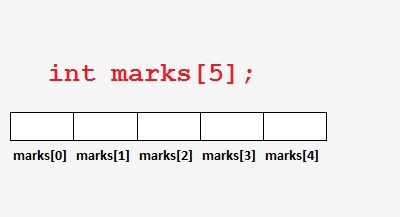# Arrays in C programming language

When we need to store multiple values of same type like names of 10 students, 50 mobile numbers, weight of 100 people. In this case, to declare and manage different variables to store separate values are really tough and unmanageable.

Then, what?

C programming language provides an amazing feature to deal with such kind of situations that is known as "Arrays".

An "Array" is a group of similar data type to store series of homogeneous pieces of data that all are same in type.

It is a derived data type which is created with the help of basic data type. An array takes contiguous memory blocks to store series of values.

There are three types of an array: 1) One Dimensional (One-D) Array, 2) Two Dimensional (Two-D) Array and 3) Multi Dimensional Array.

Here, we will understand array with One Dimensional Array.

Let’s consider a requirement, where we want to store marks of 5 students of integer type, then array declare would be:

`int marks;`

This declaration statement will define an array with 5 elements named marks, marks, ... marks.

Consider the given diagrammarks[i] will be used to access ith element, where i is the loop counter from 0 to 4.

## Array Initialization

One Dimensional Array can be initialized like this:

`int marks={98,89,77,10,34};`

or

`int marks[]={98,89,77,10,34};`

Consider the given example

```#include <stdio.h>

int main()
{
//declare & initialize array
int marks={98,89,77,10,34};
int i; //loop counter

printf("Marks are:\n");
for(i=0; i<5; i++)
printf("marks[%d]: %d\n",i,marks[i]);

printf("\n");
return 0;
}
```

Output

```marks: 98
marks: 89
marks: 77
marks: 10
marks: 34
```

### More on Arrays...

Complete array example using reading and printing with sum of all numbers:

```#include <stdio.h>
int main()
{
int num;
int i,sum;

printf("\nEnter array elements :\n");
for(i=0;i<5;i++)
{
printf("Enter elements [%d] : ",i+1);
scanf("%d",&num[i]);
}

/*sum of all numbers*/
sum=0;
for(i=0;i<5;i++)
sum+=num[i];

printf("Array elements are :\n");
for(i=0;i<5;i++)
{
printf("%d\t",num[i]);
}

printf("\nSum of all elements : %d\n",sum);

return 0;
}
```

Output

```Enter array elements :
Enter elements  : 10
Enter elements  : 20
Enter elements  : 30
Enter elements  : 40
Enter elements  : 50
Array elements are :
10	20	30	40	50
Sum of all elements : 150
```